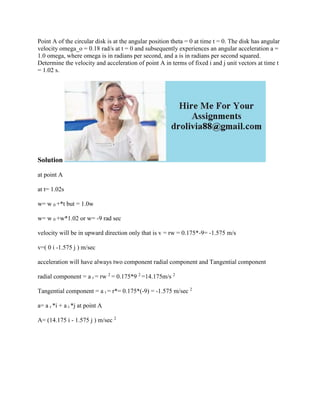Seu SlideShare está sendo baixado. ×

# Point A of the circular disk is at the angular position theta - 0 at t.docx

Anúncio
Anúncio
Anúncio
Anúncio
Anúncio
Anúncio
Anúncio
Anúncio
Anúncio
Anúncio
AnúncioCarregando em…3
×

1 de 2 Anúncio

# Point A of the circular disk is at the angular position theta - 0 at t.docx

Point A of the circular disk is at the angular position theta = 0 at time t = 0. The disk has angular velocity omega_o = 0.18 rad/s at t = 0 and subsequently experiences an angular acceleration a = 1.0 omega, where omega is in radians per second, and a is in radians per second squared. Determine the velocity and acceleration of point A in terms of fixed i and j unit vectors at time t = 1.02 s.
Solution
at point A
at t= 1.02s
w= w 0 +*t but = 1.0w
w= w 0 +w*1.02 or w= -9 rad sec
velocity will be in upward direction only that is v = rw = 0.175*-9= -1.575 m/s
v=( 0 i -1.575 j ) m/sec
acceleration will have always two component radial component and Tangential component
radial component = a r = rw 2 = 0.175*9 2 =14.175m/s 2
Tangential component = a t = r*= 0.175*(-9) = -1.575 m/sec 2
a= a r *i + a t *j at point A
A= (14.175 i - 1.575 j ) m/sec 2
.

Point A of the circular disk is at the angular position theta = 0 at time t = 0. The disk has angular velocity omega_o = 0.18 rad/s at t = 0 and subsequently experiences an angular acceleration a = 1.0 omega, where omega is in radians per second, and a is in radians per second squared. Determine the velocity and acceleration of point A in terms of fixed i and j unit vectors at time t = 1.02 s.
Solution
at point A
at t= 1.02s
w= w 0 +*t but = 1.0w
w= w 0 +w*1.02 or w= -9 rad sec
velocity will be in upward direction only that is v = rw = 0.175*-9= -1.575 m/s
v=( 0 i -1.575 j ) m/sec
acceleration will have always two component radial component and Tangential component
radial component = a r = rw 2 = 0.175*9 2 =14.175m/s 2
Tangential component = a t = r*= 0.175*(-9) = -1.575 m/sec 2
a= a r *i + a t *j at point A
A= (14.175 i - 1.575 j ) m/sec 2
.

Anúncio
Anúncio

### Point A of the circular disk is at the angular position theta - 0 at t.docx

1. 1. Point A of the circular disk is at the angular position theta = 0 at time t = 0. The disk has angular velocity omega_o = 0.18 rad/s at t = 0 and subsequently experiences an angular acceleration a = 1.0 omega, where omega is in radians per second, and a is in radians per second squared. Determine the velocity and acceleration of point A in terms of fixed i and j unit vectors at time t = 1.02 s. Solution at point A at t= 1.02s w= w 0 +*t but = 1.0w w= w 0 +w*1.02 or w= -9 rad sec velocity will be in upward direction only that is v = rw = 0.175*-9= -1.575 m/s v=( 0 i -1.575 j ) m/sec acceleration will have always two component radial component and Tangential component radial component = a r = rw 2 = 0.175*9 2 =14.175m/s 2 Tangential component = a t = r*= 0.175*(-9) = -1.575 m/sec 2 a= a r *i + a t *j at point A A= (14.175 i - 1.575 j ) m/sec 2